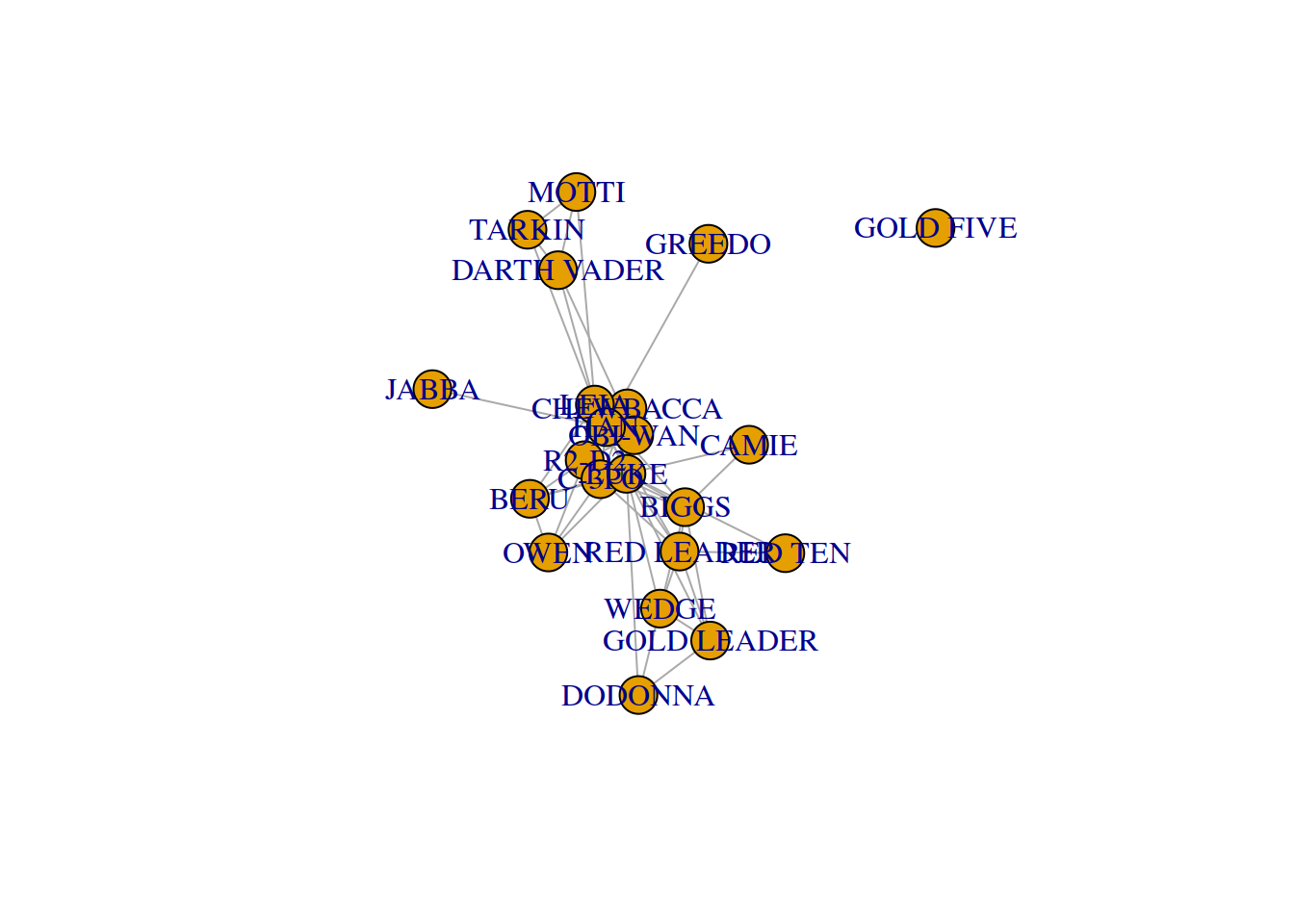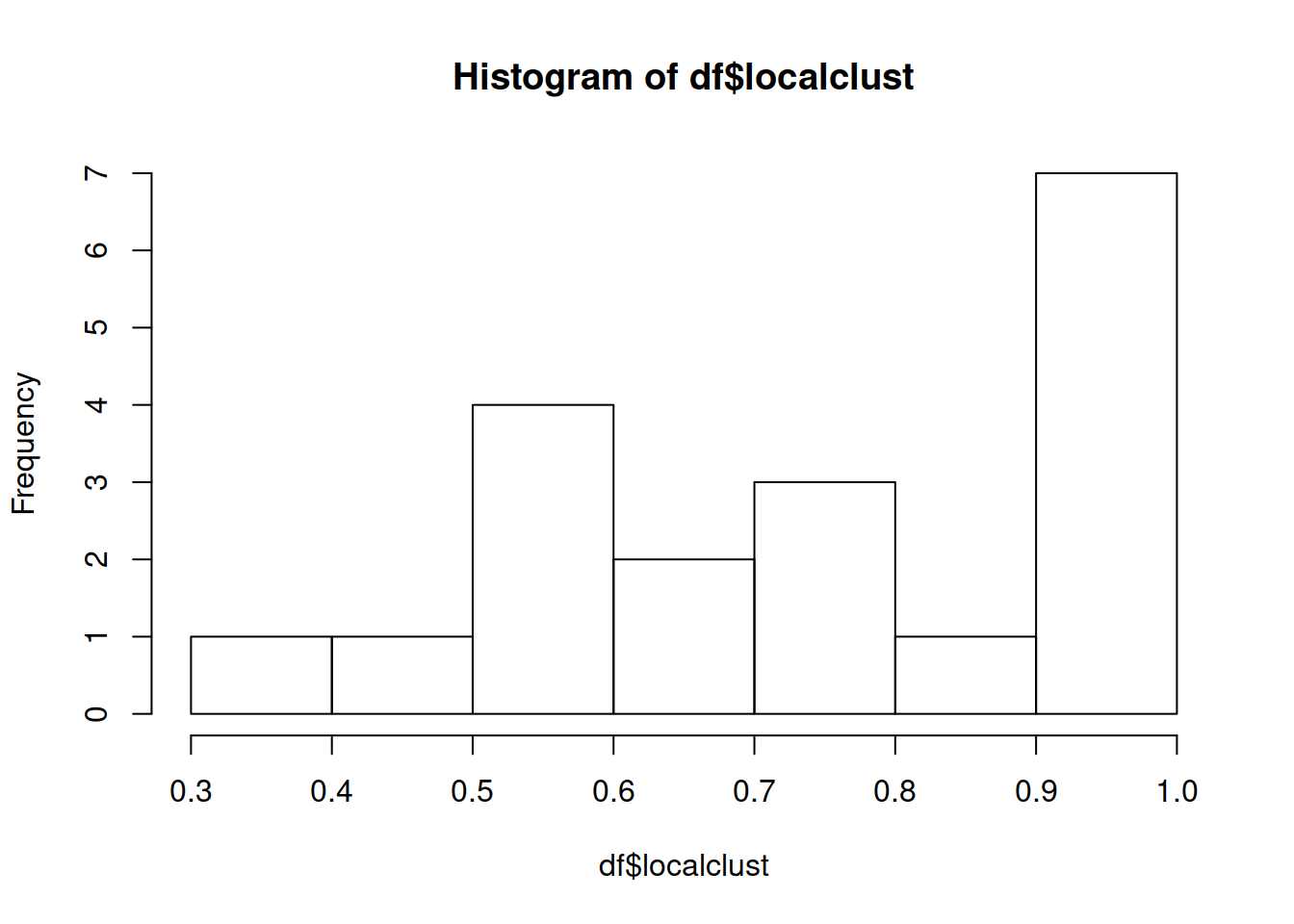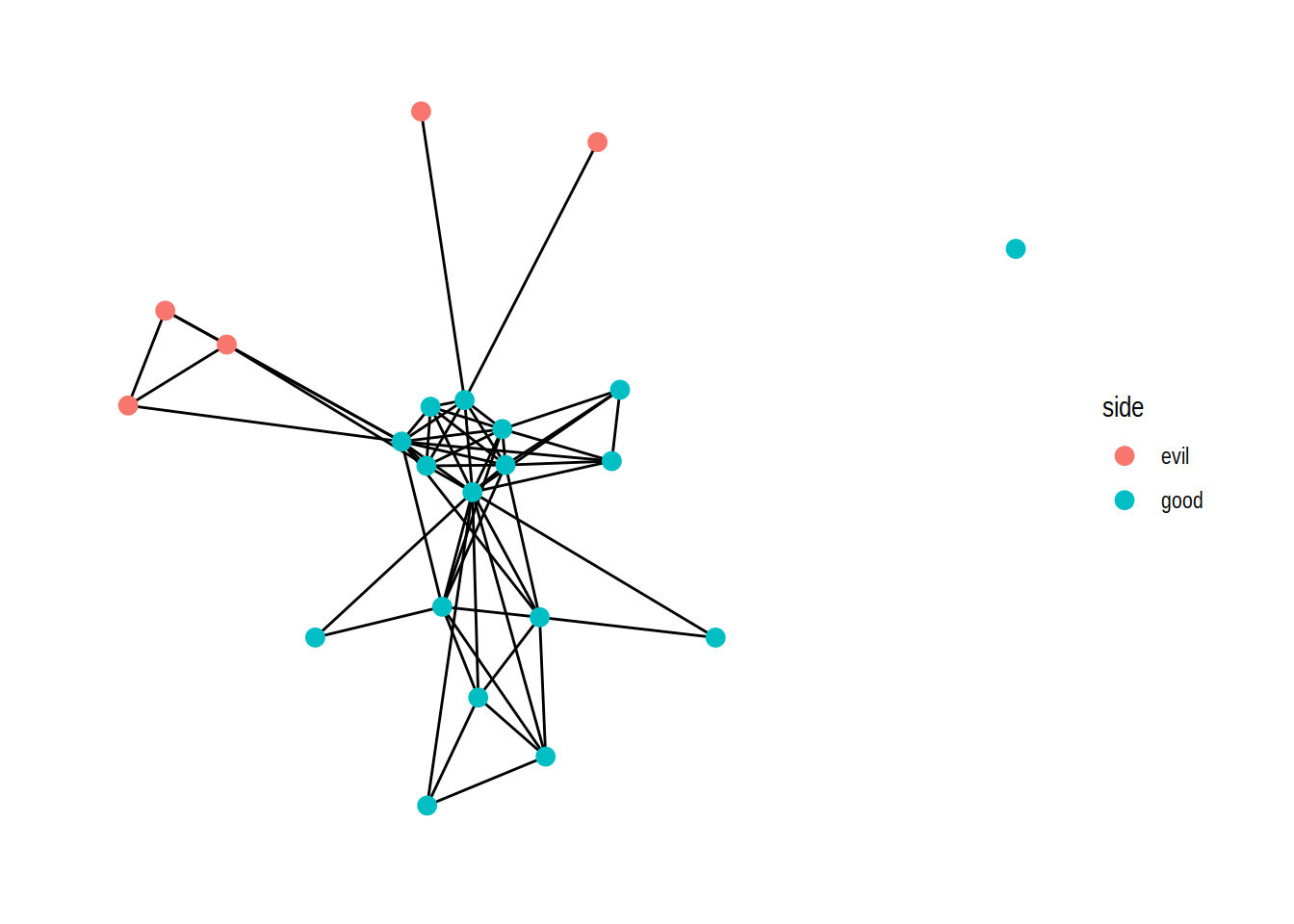In this tutorial we will learn how to analyze network data in R to measure complex metrics. As in the introduction tutorial about how to handle network data in R, we are going to use as an example the social network of interactions between the characters of Star Wars Episode IV.

### Aggregate network metrics

We start our tutorial by loading the libraries for data manipulation dplyr and tidygraph and creating the social network from data using tbl_graph:

``````library(dplyr)
library(tidygraph)
library(ggraph)

names(interactionsdf) <- c("to","from","weight") #for tbl_graph
charactersdf\$id <- seq(1:(nrow(charactersdf)))
interactionsdf\$to <- interactionsdf\$to+1
interactionsdf\$from <- interactionsdf\$from+1

starwars<-tbl_graph(charactersdf,interactionsdf, directed=F)
plot(starwars)``````Tidygraph has functions to calculate metrics on the network, they generally start with graph_ and you can see a list and their documentation if you run “?graph_measures”. They are a way to call other functions from the igraph package, you can find details about how each one is calculated in the links that you see in the help.

Using these functions in tidygraph is a bit different than how it works with other analysis packages. Tidygraph wants you to run the functions inside a pipeline, for example in a mutate call, rather than over the graph object. For example, we can measure the mean distance between all pairs of nodes in the network like this:

``````starwars %>%
mutate(mndist = graph_mean_dist()) %>%
pull(mndist) %>%
``##  1.914286``

In the code above, we call graph_mean_dist() inside the mutate function to calculate the global mean distance between all pairs of nodes. The pull command gives us the column we just calculated (mndist) and the last head simplifies the output because we would get the same number for each node.

This pipeline is a bit overcomplicated for a single network aggregate, but it is useful when we want to get local node or edge metrics and then study their distribution. For example, here we calculate the local number of triangles, which is part of the calculation we use for the clustering coefficient:

``````starwars %>%
activate(nodes) %>%
mutate(tri = local_triangles()) %>%
as_tibble() -> trianglesdf

``````## # A tibble: 6 x 4
##   name        side     id   tri
##   <fct>       <fct> <int> <dbl>
## 1 R2-D2       good      1    24
## 2 CHEWBACCA   good      2    15
## 3 C-3PO       good      3    27
## 4 LUKE        good      4    36
## 5 DARTH VADER evil      5     4
## 6 CAMIE       good      6     1``````

We can also calculate degree and filter out nodes with degree below 2 and calculate the local clustering coefficient. Since we have all values now we can plot their distribution, compute the mean, and even calculate the global clustering coefficient:

``````starwars %>%
activate(nodes) %>%
mutate(tri = local_triangles(), deg=centrality_degree()) %>%
as_tibble() %>%
filter(deg>1)-> df

df %>% mutate(localclust = 2*tri/(deg*(deg-1))) -> df

hist(df\$localclust)````````mean(df\$localclust)``
``##  0.7686945``
``2*sum(df\$tri)/sum(df\$deg*(df\$deg-1))``
``##  0.5598086``

### Assortativity

The developers of igraph have coded lots of metrics to calculate on networks, and they are accessible through tidygraph. Assortativity is one of them. Here, we take the attribute “side” of the nodes, which is set to “good” or “evil” in the movie. Using graph_assortativity(), we can calculate the assortativity coeficient of the network with respect to the side attribute:

``````starwars %>%
activate(nodes) %>%
mutate(assort = graph_assortativity(attr=side)) %>%
pull(assort) %>%
``##  0.4444444``

As you see, the network is rather assortative. We can see this if we make a plot with nodes colored according to their side:

``````starwars %>%
ggraph("fr")  + geom_edge_link()  + geom_node_point(aes(color=side), size=3) + theme_graph()``````Assortativity can also be calculated according to numeric values. For example, in the following code we calculate the degree assortativity of the network, which is similar to a correlation coefficient between the degrees of the nodes at the ends of each edge:

``````starwars %>%
activate(nodes) %>%
mutate(deg=centrality_degree()) %>%
mutate(assort = graph_assortativity(attr=deg)) %>%
pull(assort) %>%
``##  -0.1801361``

The network is a bit diassortative with respect to degree, meaning that nodes of high degree tend to be connected to nodes of lower degree, hence a bit the star-like shape of the network.

### Community detection and modularity

With tidygraph, you can also apply commmunity detection algorithms to find densely connected subgraphs. For example, you can use the Louvain algorithm, which is a fast and common way to find a division into communities that maximizes modularity:

``````starwars %>%
activate(nodes) %>%
mutate(community=as.character(group_louvain())) -> starwars

starwars %>%
activate(nodes) %>%
as_tibble()``````
``````## # A tibble: 22 x 4
##    name        side     id community
##    <fct>       <fct> <int> <chr>
##  1 R2-D2       good      1 1
##  2 CHEWBACCA   good      2 1
##  3 C-3PO       good      3 1
##  4 LUKE        good      4 2
##  5 DARTH VADER evil      5 3
##  6 CAMIE       good      6 2
##  7 BIGGS       good      7 2
##  8 LEIA        good      8 3
##  9 BERU        good      9 1
## 10 OWEN        good     10 1
## # … with 12 more rows``````

You can see the communities by drawing the network with nodes colored by community. We casted the community values to characters so they get very distinct colors in the plot:

``````starwars %>%
ggraph("fr")  + geom_edge_link()  + geom_node_point(aes(color=community), size=3) + theme_graph()``````